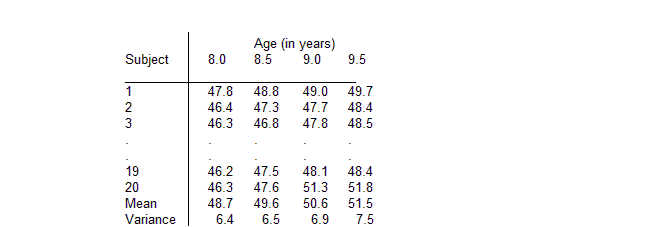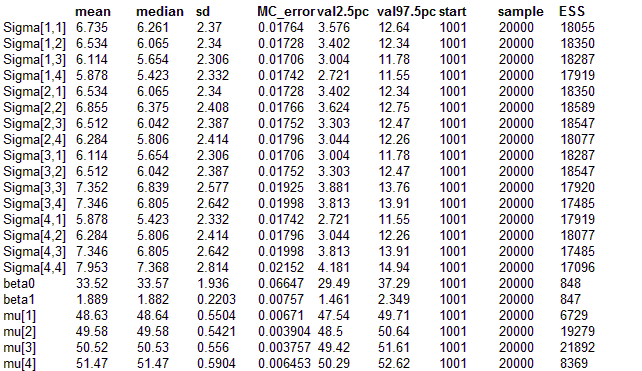#### Jaws: repeated measures analysis of variance

Elston and Grizzle (1962) present repeated measurements of ramus (jaw) bone height on a
cohort of 20 boys over an 18 month period:Interest focuses on describing the average growth curve of the ramus bone. The 4
measurements Yi = {Yi1, Yi2, Yi3, Yi4} for each child i are assumed to be correlated and follow a multivariate normal (MVN) distribution with unknown population mean vector μ and precision matrix Ω. That is Yi ~ MVN(μ, Ω)

The following location models for the population mean μ were fitted in turn:
E(μi)   =   β0   Constant height
E(μi)   =   β0 + β1 xj   Linear growth curve
E(μi)   =   β0 + β1 xj + β2 xj2    Quadratic growth curve

where xj = age at jth measurement. Non-informative independent normal priors were specified for the regression coefficients β0, β1, and β2. The population precision matrix Ω was assumed to follow a Wishart(R, ρ) distribution. To represent vague prior knowledge, we chose the the degrees of freedom ρ for this distribution to be as small as possible (i.e. 4, the rank of Ω). The scale matrix R was specified as a 4x4 diag(1) matrix which represents an assessment of the order of magnitude of the covariance matrix Ω-1 for Yi (see subsection on the use of the Wishart distribution in the "Multivariate normal nodes'' section of the Classic BUGS manual (version 0.50). Note that except for cases with very few individuals, the choice of R has little effect on the posterior estimate of Ω-1 (Lindley, 1970).
BUGS language for the Jaws example
model
{
beta0 ~ dnorm(0.0, 0.001)
beta1 ~ dnorm(0.0, 0.001)
for (i in 1:N) {
Y[i, 1:M] ~ dmnorm(mu[], Omega[ , ])
}
for(j in 1:M) {
mu[j] <- beta0 + beta1* age[j]
}
Omega[1 : M , 1 : M] ~ dwish(R[ , ], 4)
Sigma[1 : M , 1 : M] <- inverse(Omega[ , ])

}

##### Data
```    list(M = 4, N = 20, Y = structure(      .Data = c(47.8, 48.8, 49.0, 49.7,               46.4, 47.3, 47.7, 48.4,               46.3, 46.8, 47.8, 48.5,               45.1, 45.3, 46.1, 47.2,               47.6, 48.5, 48.9, 49.3,               52.5, 53.2, 53.3, 53.7,               51.2, 53.0, 54.3, 54.5,               49.8, 50.0, 50.3, 52.7,               48.1, 50.8, 52.3, 54.4,               45.0, 47.0, 47.3, 48.3,               51.2, 51.4, 51.6, 51.9,               48.5, 49.2, 53.0, 55.5,               52.1, 52.8, 53.7, 55.0,               48.2, 48.9, 49.3, 49.8,               49.6, 50.4, 51.2, 51.8,               50.7, 51.7, 52.7, 53.3,               47.2, 47.7, 48.4, 49.5,               53.3, 54.6, 55.1, 55.3,               46.2, 47.5, 48.1, 48.4,               46.3, 47.6, 51.3, 51.8),            .Dim = c(20, 4)),   age = c(8.0, 8.5, 9.0, 9.5),       R = structure(            .Data = c(1, 0, 0, 0,                      0, 1, 0, 0,                      0, 0, 1, 0,                      0, 0, 0, 1), .            Dim = c(4, 4))) ```
##### Inits for chain 1
``` list(beta0 = 40, beta1 = 1) ```

##### Inits for chain 2
``` list(beta0 = 10, beta1 = 10) ```
ResultsMetropolis results

mean   median   sd   MC_error   val2.5pc   val97.5pc   start   sample   ESS
Sigma[1,1]   6.705   6.259   2.359   0.02164   3.546   12.49   5001   200000   11891
Sigma[1,2]   6.506   6.072   2.335   0.02182   3.383   12.28   5001   200000   11454
Sigma[1,3]   6.077   5.643   2.299   0.02331   2.98   11.76   5001   200000   9731
Sigma[1,4]   5.833   5.387   2.308   0.0243   2.718   11.56   5001   200000   9023
Sigma[2,1]   6.506   6.072   2.335   0.02182   3.383   12.28   5001   200000   11454
Sigma[2,2]   6.826   6.376   2.405   0.02304   3.601   12.64   5001   200000   10894
Sigma[2,3]   6.474   6.015   2.383   0.0245   3.273   12.35   5001   200000   9457
Sigma[2,4]   6.238   5.784   2.393   0.0254   3.023   12.21   5001   200000   8876
Sigma[3,1]   6.077   5.643   2.299   0.02331   2.98   11.76   5001   200000   9731
Sigma[3,2]   6.474   6.015   2.383   0.0245   3.273   12.35   5001   200000   9457
Sigma[3,3]   7.301   6.795   2.57   0.02809   3.874   13.72   5001   200000   8371
Sigma[3,4]   7.281   6.766   2.62   0.02936   3.792   13.78   5001   200000   7967
Sigma[4,1]   5.833   5.387   2.308   0.0243   2.718   11.56   5001   200000   9023
Sigma[4,2]   6.238   5.784   2.393   0.0254   3.023   12.21   5001   200000   8876
Sigma[4,3]   7.281   6.766   2.62   0.02936   3.792   13.78   5001   200000   7967
Sigma[4,4]   7.873   7.322   2.778   0.0316   4.171   14.74   5001   200000   7728
beta0   33.62   33.63   1.932   0.02356   29.8   37.43   5001   200000   6723
beta1   1.877   1.876   0.2196   0.002676   1.444   2.312   5001   200000   6733
mu   48.64   48.64   0.55   0.002502   47.55   49.72   5001   200000   48317
mu   49.58   49.58   0.5414   0.001547   48.51   50.65   5001   200000   122492
mu   50.52   50.52   0.5548   0.001451   49.42   51.61   5001   200000   146265
mu   51.46   51.46   0.5887   0.002323   50.29   52.62   5001   200000   64223